# Logic Operations

 Tip An easy way to get a sense of how artificial neural networks work is by simulating the basic logic operations: AND, OR, NOT and XOR.

 Problem 1 The OR operation is used in logic circuits and its symbol is shown below. In its more basic form, it has two inputs and one output. For each input in the table, compute manually the output of the ANN shown below. Assume that the activation function in the network is f(y) = tanh(1.5 y).

 x1 x2 z1 0 0 0 1 1 0 1 1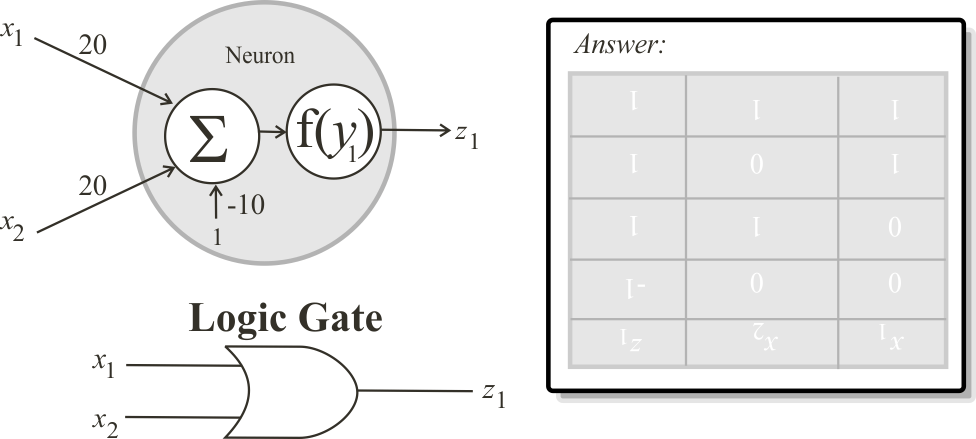Problem 2 Repeat the last problem using Neural Lab.

 Solution 2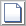New Project click the button to create a new project called Or and write the code shown below.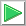Run click the button to execute the code. If you do not have any errors, the variables x, W and z will be displayed in the variable list. Click on z to review your results.

 Or\Main.lab LayerNet net; net.Create(2, 0, 0, 1); //________ Create and set the ANN weights Matrix w; w.Create(1, 3); w = 20; w = 20; w = -10; net.SetWeights(3, w); //Layer Output Index = 3 //________ Create the input Matrix x; x.Create(4, 2); x = 0; x = 0; x = 0; x = 1; x = 1; x = 0; x = 1; x = 1; Matrix z = net.Run(x);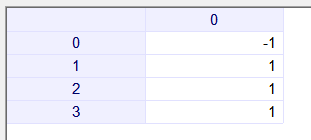## Matlab

Matlab is a high-level language and interactive environment for numerical computation, visualization, and programming developed by MathWorks. Matlab includes a toolbox for artificial neural networks than can be open by executing the command nntool from the command window in Matlab. The figure below shows the nntool that may appear different in other versions of Matlab. Note that the line net.IW the letter after the dot is a "capitol i".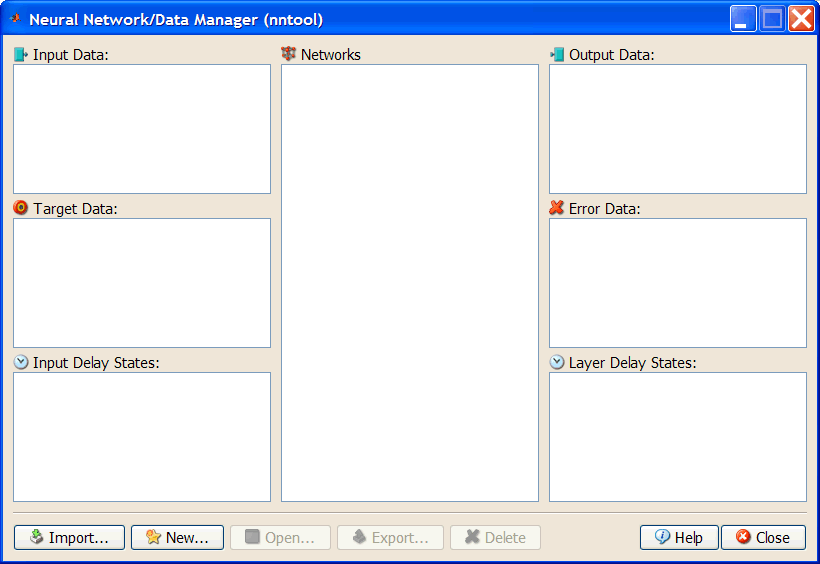Problem 3 Repeat the previous problem using Matlab. First open Matlab. Second, create the my_or.m file as shown below. Finally, change the location of the working folder in Matlab, and execute the m-file.

 my_or.m clear; input = [0, 0, 1, 1; 0, 1, 0, 1]; target = [0, 1, 1, 1]; net = newff([0 1; 0 1], 1, {'logsig'}); net.IW{1, 1} = [20, 20]; net.b{1} = -10; output = sim(net, input)

 Output > > my_or output =     0.0000    1.0000    1.0000    1.0000

 Problem 4 The AND operation is also used in logic circuits and its symbol is shown below. In its more basic form, it has two inputs and one output. For each input in the table, compute manually the output of the ANN shown below. Assume that the activation function in the network is f(y) = tanh(1.5 y).

 x1 x2 z1 0 0 0 1 1 0 1 1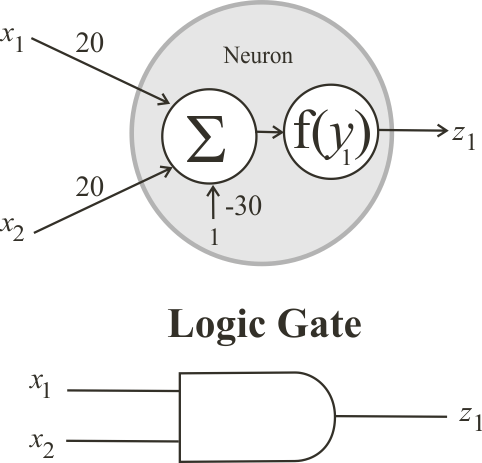Problem 5 Repeat the last problem using Neural Lab. Your project must be called And.

 Problem 6 Compute by trial and error the weights of the ANN to produce the logic NOT operation as described in the table. Suppose that the action function is f(x) = logsig(x)

 x1 z1 0 1 1 0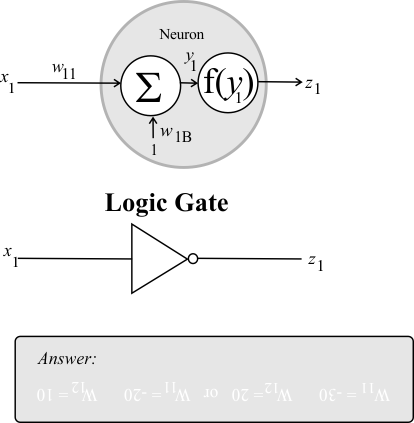Problem 7 Repeat the last problem using Neural Lab. In Neural Lab the activation function is f(x) = tanh(x), thus

 x1 z1 0 1 1 -1

 Hint Generate randomly the two weights until the desired output is obtained when the specified input is applied. Use the control execution sentence: while. Suppose that the weights are in the range from -30 to 30.

 Solution 7New Project click the button to create a new project called Not and write the code shown below.Run click the button to execute the code. If you do not have any errors, the variables x, W and z will be displayed in the variable list. Click on W and z to verify the results.

 Not.lab LayerNet net; net.Create(1, 0, 0, 1); //________ Create the ANN weights Matrix w; w.Create(1, 2); //________ Create the input Matrix x; x.Create(2, 1); x = 0; x = 1; Matrix z; double error = 100.0; while (error > 0.01) {      ...      net.SetWeights(3, w); //Layer Output Index = 3      z = net.Run(x);      ... }